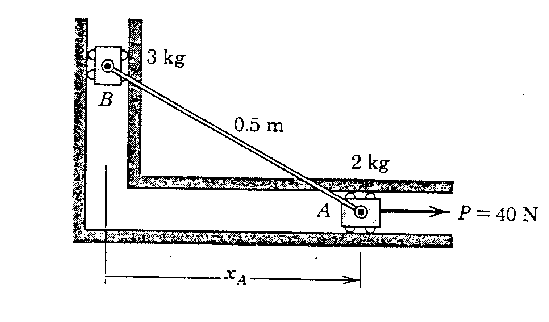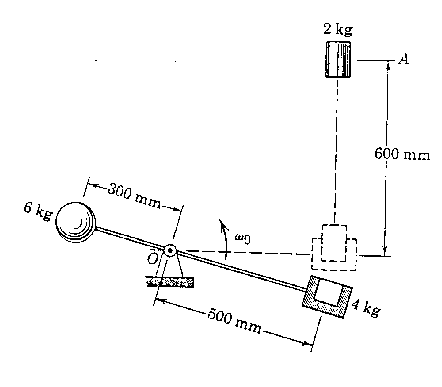Engineering Dynamics (EngM 373) -- Exam II -- Spring 1996

Note to the students: The following is a typical "Exam I" for dynamics. It should give you an idea of the difficulty of the exam. Try to do the exam in 50 minutes without the aid of your book or your notes, only using your one page of equations (no solutions to problems). For each problem, first layout the procedure to obtain the desired solution, then complete the problem by doing all the details. Don't forget to draw free-body diagrams for the problems requiring a force analysis, and to present your result in three significant digits with proper units. If the figures are not clear, you can find them in the book.

Problem # 1: The sliders A and B are connected by the light rigid bar of length l=0.5 m and move with negligible friction in the horizontal slots shown. For the position when xA = 0.4 m, the velocity of A is vA = 0.9 m/s to the right. Determine the acceleration of each slider and the force in the bar at this instant.

(10 points)Problem # 2: The Spring has an unstretched length of 25 in. If the system is released from rest in the position shown, determine the speed v of the ball when it has dropped a vertical distance of 10 in.

(10 points)Problem # 3: The 6-kg sphere and the 4-kg block (shown in section) are secured to the arm of negligible mass that rotates in the vertical plane about a horizontal axis at O. The 2-kg plug is released from rest at A and falls into the recess in the block when the arm has reached the horizontal position. An instant before engagement, the arm has an angular velocity o=2 rad/s. Determine the angular velocity of the arm immediately after the plug has wedged itself in the block.

(10 points)For extra credit: Start from Newton's 2nd law and derive the work-energy relation.

(3 points)

For extra credit: Show that the work done by a conservative force is negative the change in its potential energy.

(3 points)